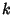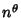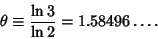## Stolarsky-Harborth Constant

N.B. A detailed on-line essay by S. Finch was the starting point for this entry.

Letbe the number of 1s in the Binary expression of. Then the number of Odd Binomial Coefficientswhereis(Glaisher 1899, Fine 1947). The number of Odd elements in the firstrows of Pascal's Triangle is(1)

This function is well approximated by, where(2)

Stolarsky and Harborth showed that(3)

The value(4)

is called the Stolarsky-Harborth constant.

References

Finch, S. Favorite Mathematical Constants.'' http://www.mathsoft.com/asolve/constant/stlrsky/stlrsky.html

Fine, N. J. Binomial Coefficients Modulo a Prime.'' Amer. Math. Monthly 54, 589-592, 1947.

Wolfram, S. Geometry of Binomial Coefficients.'' Amer. Math. Monthly 91, 566-571, 1984.